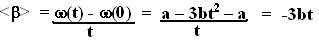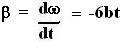## Tuesday, July 24, 2007

### Irodov Problem 1.46

(a) The average angular velocity is simply the angle traversed by the time it took to traverse this angle and thus is given by,The particle stops when its angular velocity is 0, thus it stops at time given by,Thus, the mean angular velocity will be,The mean angular acceleration is the amount of angular velocity gained by the time it took to gain it. This is given by,Thus, when the particle stops, its mean angular acceleration will be,(b) The angular acceleration at any given time is the derivative of its angular velocity given by,Thus, when the particle stops it will be,